Second Law Of Thermodynamic MCQ Level – 1 (Part - 2)

# Second Law Of Thermodynamic MCQ Level – 1 (Part - 2)

Test Description

## 10 Questions MCQ Test Topic wise Tests for IIT JAM Physics | Second Law Of Thermodynamic MCQ Level – 1 (Part - 2)

Second Law Of Thermodynamic MCQ Level – 1 (Part - 2) for Physics 2023 is part of Topic wise Tests for IIT JAM Physics preparation. The Second Law Of Thermodynamic MCQ Level – 1 (Part - 2) questions and answers have been prepared according to the Physics exam syllabus.The Second Law Of Thermodynamic MCQ Level – 1 (Part - 2) MCQs are made for Physics 2023 Exam. Find important definitions, questions, notes, meanings, examples, exercises, MCQs and online tests for Second Law Of Thermodynamic MCQ Level – 1 (Part - 2) below.
Solutions of Second Law Of Thermodynamic MCQ Level – 1 (Part - 2) questions in English are available as part of our Topic wise Tests for IIT JAM Physics for Physics & Second Law Of Thermodynamic MCQ Level – 1 (Part - 2) solutions in Hindi for Topic wise Tests for IIT JAM Physics course. Download more important topics, notes, lectures and mock test series for Physics Exam by signing up for free. Attempt Second Law Of Thermodynamic MCQ Level – 1 (Part - 2) | 10 questions in 30 minutes | Mock test for Physics preparation | Free important questions MCQ to study Topic wise Tests for IIT JAM Physics for Physics Exam | Download free PDF with solutions
 1 Crore+ students have signed up on EduRev. Have you?
Second Law Of Thermodynamic MCQ Level – 1 (Part - 2) - Question 1

### T-S diagram for Carnot cycle is. Select one:

Detailed Solution for Second Law Of Thermodynamic MCQ Level – 1 (Part - 2) - Question 1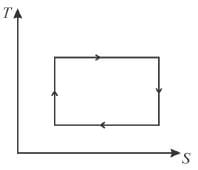Second Law Of Thermodynamic MCQ Level – 1 (Part - 2) - Question 2

### For the thermodynamic process shown in the figure, corresponding T-S diagram is.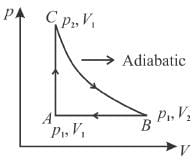Select one:

Detailed Solution for Second Law Of Thermodynamic MCQ Level – 1 (Part - 2) - Question 2

Process C to B is adiabatic
it is is isentropic process.
Hence, (b) and (d) cannot be correct.
B to A is isobaric process but in option (c), B to A is isothermal.
Hence (c) is also not correct.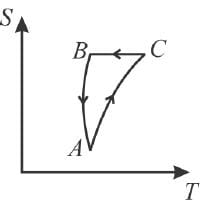Second Law Of Thermodynamic MCQ Level – 1 (Part - 2) - Question 3

### All natural processes are irreversible. This is a direct consequence of. Select one:

Detailed Solution for Second Law Of Thermodynamic MCQ Level – 1 (Part - 2) - Question 3

An irreversible processes increases the entropy of the universe. However, because entropy is a state function, the change in entropy of the system is same whether the process is reversible or irreversible.
All the natural processes are irreversible. This irreversibility results from the fact that if a thermodynamic system, which is any system of  sufficient complexity, of interacting molecules is brought from one thermodynamic state to another, the configuration or arrangement of the atoms and molecules in the system will change in a which that is not easily predictable. During this transformation, there will be a certain amount of heat energy loss or dissipation due to intermolecular friction and collision, energy  that will not be recoverable if the process is reversed.
The correct answer is: Second law of thermodynamics

Second Law Of Thermodynamic MCQ Level – 1 (Part - 2) - Question 4

In a refrigerator, the heat exhausted to the outer atmosphere is.
Select one:

Detailed Solution for Second Law Of Thermodynamic MCQ Level – 1 (Part - 2) - Question 4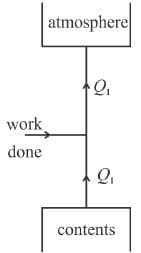Q1 = W + Q2
Hence   Q1 > Q2.
The correct answer is: more than that absorbed from the contents

Second Law Of Thermodynamic MCQ Level – 1 (Part - 2) - Question 5

A given amount of heat cannot be completely converted into work. However, it is possible to convert a given amount of work completely in heat. This statement results from the
Select one:

Detailed Solution for Second Law Of Thermodynamic MCQ Level – 1 (Part - 2) - Question 5

The above statement comes from Kelvin-Plank’s statement of second law of thermodynamics.
The correct answer is: Second law of thermodynamics

Second Law Of Thermodynamic MCQ Level – 1 (Part - 2) - Question 6

The temperature of the surface of the sun is approximately 6000K. It we take a big lens and focus the sun rays and produce a temperature of 8000K. This will violate
Select one:

Detailed Solution for Second Law Of Thermodynamic MCQ Level – 1 (Part - 2) - Question 6

The creation of a temperature of 8000K by transferring heat from a colder body (sun at 6000K) by means of a lens is a violation of second law of thermodynamics.
The correct answer is: Second law

Second Law Of Thermodynamic MCQ Level – 1 (Part - 2) - Question 7

The area of the Carnot cycle on a T-S diagram represents
Select one:

Detailed Solution for Second Law Of Thermodynamic MCQ Level – 1 (Part - 2) - Question 7

The total area under top side of rectangle is total heat absorbed. The area under bottom side is total heat released. The area inside the rectangle is total heat absorbed- total heat released = total work done in a cycle.”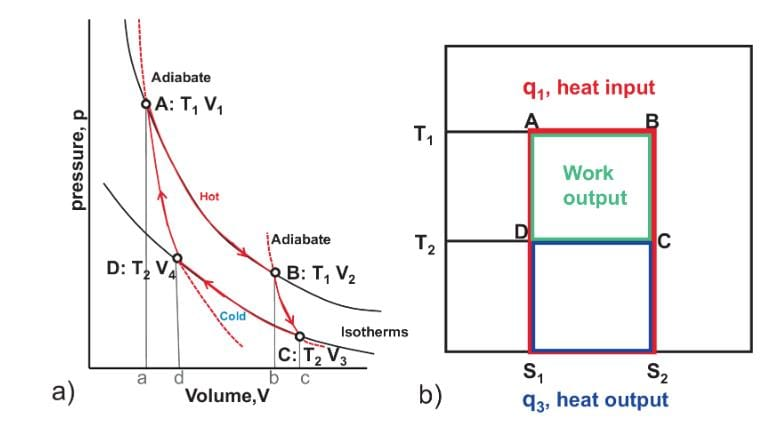Second Law Of Thermodynamic MCQ Level – 1 (Part - 2) - Question 8

For any process, the second law of thermodynamics requires that the change of entropy of universe is.
Select one:

Detailed Solution for Second Law Of Thermodynamic MCQ Level – 1 (Part - 2) - Question 8

From second law thermodynamics,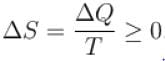The correct answer is: positive or zero

Second Law Of Thermodynamic MCQ Level – 1 (Part - 2) - Question 9

For a reversible cyclic process, the value of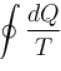is.
Select one:

Detailed Solution for Second Law Of Thermodynamic MCQ Level – 1 (Part - 2) - Question 9

For a reversible cycle process just like the Carnot engine,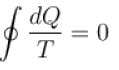This is because any reversible cycle can be represented as a series of miniature Carnot cycle.
The correct answer is: always equal to zero

Second Law Of Thermodynamic MCQ Level – 1 (Part - 2) - Question 10

In a heat engine maximum heat that can be converted into mechanical work.
Select one:

Detailed Solution for Second Law Of Thermodynamic MCQ Level – 1 (Part - 2) - Question 10

Since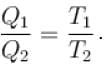The work temperature plays an important role in the efficiency of the engine.
The correct answer is: depends upon working temperature

## Topic wise Tests for IIT JAM Physics

217 tests
 Use Code STAYHOME200 and get INR 200 additional OFF Use Coupon Code
Information about Second Law Of Thermodynamic MCQ Level – 1 (Part - 2) Page
In this test you can find the Exam questions for Second Law Of Thermodynamic MCQ Level – 1 (Part - 2) solved & explained in the simplest way possible. Besides giving Questions and answers for Second Law Of Thermodynamic MCQ Level – 1 (Part - 2), EduRev gives you an ample number of Online tests for practice

217 tests